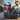# Prime Factors Kata in Clojure

June 22, 2012

I’m learning Clojure and thought doing the Prime Factors Kata would be a good way to dive in. For those unfamiliar with the Kata:

Write a static method primefactors() that, given an integer N, will return a list of integers such that the numbers are the prime factors of N and are arranged in increasing numerical order. primefactors(1) should return an empty list. ―Uncle Bob

Coming from a C++ and Python background, it was a bumpy ride. Having a function return the result of its last operation was the biggest hangup for me. I kept wanting to introduce values (aka variables) and make my functions do too many things but it just wouldn’t let me! The steps I took follow. Each is separated by a horizontal line with changes from the previous iteration in red. The prime factors of four caused modifications to the algorithm that allowed the next two tests to pass without further changes. It was test-driven using the Midje unit testing framework.

`.css-1baulvz{display:inline-block;}(fact (primefactors 1) => (list))`
`(defn primefactors [n]  (list))`

`(fact (primefactors 2) => (list 2))`
`(defn primefactors [n]  (if (> n 1)    (list 2)    (list)  ))`

`(fact (primefactors 3) => (list 3))`
`(defn primefactors [n]  (if (> n 1)    (list n)    (list)  ))`

`(fact (primefactors 4) => (list 2 2))`
`(defn primefactors  ([n] (primefactors n 2))  ([n candidate]    (cond (<=n 1) (list)      >(= 0 (rem n candidate)) (conj (primefactors (/ n candidate)) candidate)      :else (list n))  ))`

`(fact (primefactors 6) => (list 2 3))`

`(fact (primefactors 8) => (list 2 2 2))`

`(fact (primefactors 9) => (list 3 3))`
`(defn primefactors  ([n] (primefactors n 2))  ([n candidate]    (cond (<= n 1) (list)          (= 0 (rem n candidate)) (conj (primefactors (/ n candidate)) candidate)          :else (primefactors n (inc candidate))    )  ))`

The end result is the last code snippet and all seven tests. It’s available on Github.Rocky Warren's blog.

Principal Engineer and Architect at Vertex Software. I do other stuff too.

PhotosResumeNotes